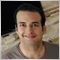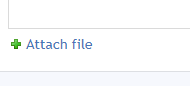# turning mql4 indicator to mql5 version251

hello,

i want to make a mql5 version of this indicator but i can, i think candlecheck functions not working but i don't know why, they work in mql4.

```#property strict
#property indicator_chart_window
#property indicator_buffers 2
#property indicator_color1 Blue
#property indicator_color2 Red

input int X_Candles=3;
input int ArrowSize=1;

double up[],down[];
datetime TimeStamp;
//+------------------------------------------------------------------+
//| Custom indicator initialization function                         |
//+------------------------------------------------------------------+
int OnInit()
{
SetIndexLabel(0,"UpCross");
SetIndexLabel(1,"DownCross");
SetIndexBuffer(0,up);
SetIndexBuffer(1,down);
SetIndexStyle(0,DRAW_ARROW,0,ArrowSize);
SetIndexStyle(1,DRAW_ARROW,0,ArrowSize);
SetIndexArrow(0, 233);
SetIndexArrow(1, 234);
return(INIT_SUCCEEDED);
}
//+------------------------------------------------------------------+
//| Custom indicator iteration function                              |
//+------------------------------------------------------------------+
int OnCalculate(const int rates_total,
const int prev_calculated,
const datetime &time[],
const double &open[],
const double &high[],
const double &low[],
const double &close[],
const long &tick_volume[],
const long &volume[],
{

int shift;
int counted_bars = IndicatorCounted();
if(counted_bars < 0)  return(-1);
if(counted_bars > 0)   counted_bars--;
int limit = Bars-counted_bars;
if(counted_bars==0) limit-=1+1;
for(shift=1;shift<=limit-X_Candles;shift++)
{
up[shift]= EMPTY_VALUE;
down[shift]=EMPTY_VALUE;

if(candlecheck_sell(shift) && Close[shift]<Open[shift])
down[shift]=iHigh(Symbol(),0,shift)+20*MathPow(10,-Digits);
up[shift]=iLow(Symbol(),0,shift)-20*MathPow(10,-Digits);

}
return(rates_total);
}
//+------------------------------------------------------------------+
{
bool result=false;
for(int j=candle+X_Candles ; j>=candle+1 ; j--)
{
if(Close[j]<Open[j])
result=true;
else
{
result=false;
break;
}
}
return(result);
}
//+------------------------------------------------------------------+
bool candlecheck_sell(int candle)
{
bool result=false;
for(int j=candle+X_Candles ; j>=candle+1 ; j--)
{
if(Close[j]>Open[j])
result=true;
else
{
result=false;
break;
}
}
return(result);
}
//+------------------------------------------------------------------+```

```#property strict
#property indicator_chart_window
//--
#property indicator_buffers 2
#property indicator_plots 2
//--
#property indicator_label1  "UpCross"
#property indicator_type1   DRAW_ARROW
#property indicator_color1  clrBlue
#property indicator_width1  1
//--
#property indicator_label2  "DownCross"
#property indicator_type2   DRAW_ARROW
#property indicator_color2  clrRed
#property indicator_width2  1
//
input int X_Candles=9;
input int ArrowSize=1;

double down[], up[];

MqlRates rate[];

//+------------------------------------------------------------------+
//| Custom indicator initialization function                         |
//+------------------------------------------------------------------+
int OnInit()
{
SetIndexBuffer(0,up);
SetIndexBuffer(1,down);

//---
//--- Define the symbol code for drawing in PLOT_ARROW
PlotIndexSetInteger(0,PLOT_ARROW,233);
//--- Set the vertical shift of arrows in pixels
PlotIndexSetInteger(0,PLOT_ARROW_SHIFT,5);
//--- Set as an empty value 0
PlotIndexSetDouble(0,PLOT_EMPTY_VALUE,0);

//--- Define the symbol code for drawing in PLOT_ARROW
PlotIndexSetInteger(1,PLOT_ARROW,234);
//--- Set the vertical shift of arrows in pixels
PlotIndexSetInteger(1,PLOT_ARROW_SHIFT,5);
//--- Set as an empty value 0
PlotIndexSetDouble(1,PLOT_EMPTY_VALUE,0);

return(INIT_SUCCEEDED);
}

//+------------------------------------------------------------------+
//| Custom indicator iteration function                              |
//+------------------------------------------------------------------+
int OnCalculate(const int rates_total,
const int prev_calculated,
const datetime &time[],
const double &open[],
const double &high[],
const double &low[],
const double &close[],
const long &tick_volume[],
const long &volume[],
{
//---
//----------------------------arrow drawing--------------------------+

ArraySetAsSeries(rate, true);
CopyRates(_Symbol,PERIOD_CURRENT, 0, WHOLE_ARRAY, rate);

int total=0;
//--- if it is the first call
if(prev_calculated==0)
{
//--- set zero values to zero indexes
total=rates_total-1;
}
else
{
total=rates_total-prev_calculated;
}

for(int i=1;i<=total-X_Candles;i++)
{
//--
if(candlecheck_sell(i) && close[i]<open[i])
{
down[i]=high[i]+20*MathPow(10,-_Digits);
}
//--- Otherwise specify the zero value

//--
{

up[i]=low[i]-20*MathPow(10,-_Digits);
}

}

return(rates_total);
}

//+------------------------------------------------------------------+
{
bool result=false;
for(int j=candle+X_Candles ; j>=candle+1 ; j--)
{
if(rate[j].close<rate[j].open)
result=true;
else
{
result=false;
break;
}
}
return(result);
}
//+------------------------------------------------------------------+
bool candlecheck_sell(int candle)
{
bool result=false;
for(int j=candle+X_Candles ; j>=candle+1 ; j--)
{
if(rate[j].close>rate[j].open)
result=true;
else
{
result=false;
break;
}
}
return(result);
}
//+------------------------------------------------------------------+

```Moderator
160231

Hossein Zandi :

hello,

i want to make a mql5 version of this indicator but i can, i think candlecheck functions not working but i don't know why, they work in mql4.

Nothing is clear. Just a bunch of text.

PAY ATTENTION: the rules of good form say: in the code ALWAYS SHOULD BE A HAT! Example:

```//+------------------------------------------------------------------+
//|                                                  Accelerator.mq5 |
//|                   Copyright 2009-2017, MetaQuotes Software Corp. |
//|                                              http://www.mql5.com |
//+------------------------------------------------------------------+
#property copyright   "2009-2017, MetaQuotes Software Corp."